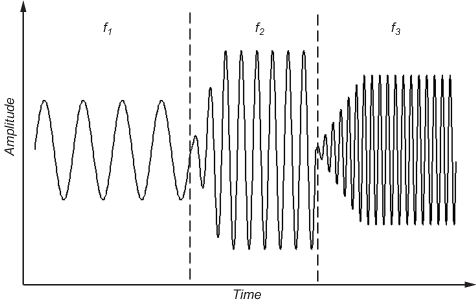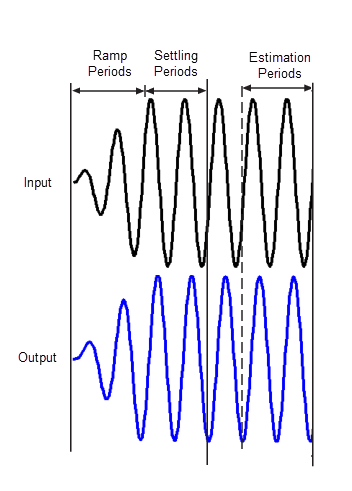Main Content

## Sinestream Input Signals

In frequency response estimation, a sinestream signal consists of sine waves of varying frequencies applied one after another. Each frequency excites the system for a period of time.You can use a sinestream input signal for estimation at the command line, in Model Linearizer, or with the Frequency Response Estimator block. The estimation algorithm injects the sinestream signal at the input point you specify for estimation, and measures the response at the output point.Sinestream signals are recommended for most situations. They are especially useful when your system contains strong nonlinearities or you require highly accurate frequency response models. The frequency-response model that results when you use a sinestream input contains all the frequencies in the sinestream signal.

When you create your sinestream input signal, you specify the following parameters for each frequency:

• Number of periods for ramping up the signal to its maximum value

• Number of settling periods

• Total number of periods.

The number of estimation periods is the total number of periods minus the number of settling periods. The estimation algorithms discard response data collected during the ramp periods and settling periods time frames. Doing so allows any transient responses to die out. The algorithm uses data collected during the estimation periods to compute the estimated frequency response.(In offline estimation, if FIR filtering is on, the software also discards the first estimation period as shown in the illustration. For details about the offline and online estimation algorithms, see the Algorithms sections of `frestimate` and Frequency Response Estimator, respectively.)

### Create Sinestream Signals Using Model Linearizer

In the Model Linearizer, to use a sinestream input signal for estimation, on the Estimation tab, select:

• Input Signal > Sinestream when the sample time of the I/Os is continuous.

• Input Signal > Fixed Sample Time Sinestream when the sample time of the I/Os is discrete.

You can specify the frequencies to use in the sinestream in one of two ways:

Other parameters you can specify for a sinestream signal in Model Linearizer include:

• Amplitude — Amplitude of injected sine waves

• Number of periods — Total number of periods at each frequency

• Settling periods — Number of periods to discard for the estimation computation

• Ramp periods — Number of periods for ramping up the amplitude of each sine wave to its maximum value

• Perform filtering to improve estimation results — Filter the response data before estimating frequency response (see the Algorithms section of `frestimate`)

### Create Sinestream Signals Using MATLAB Code

To create a sinestream signal for estimation at the command line with `frestimate`, use:

### Sinestream Signals for Online Estimation

You can use a sinestream signal for online estimation with the Frequency Response Estimator block. To do so, set the Experiment mode parameter to Sinestream. Other relevant block parameters include:

• Frequencies — Vector of frequencies for the sinestream signal.

• Amplitudes — Signal amplitudes. You can specify a single amplitude for all frequencies, or separate amplitudes for each frequency.

• Number of settling periods — Number of periods to discard for the estimation computation.

• Number of estimation periods — Number of periods to use in the estimation computation.

For details, see the Frequency Response Estimator block reference page.

## SupportGet trial now